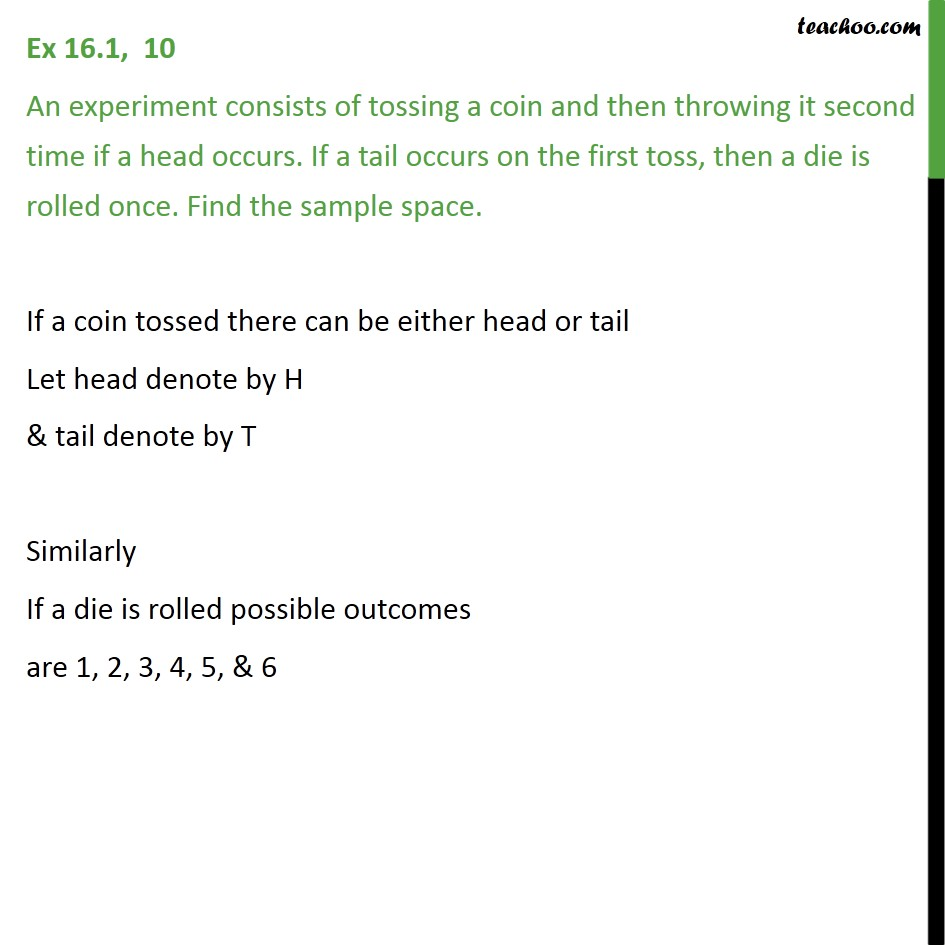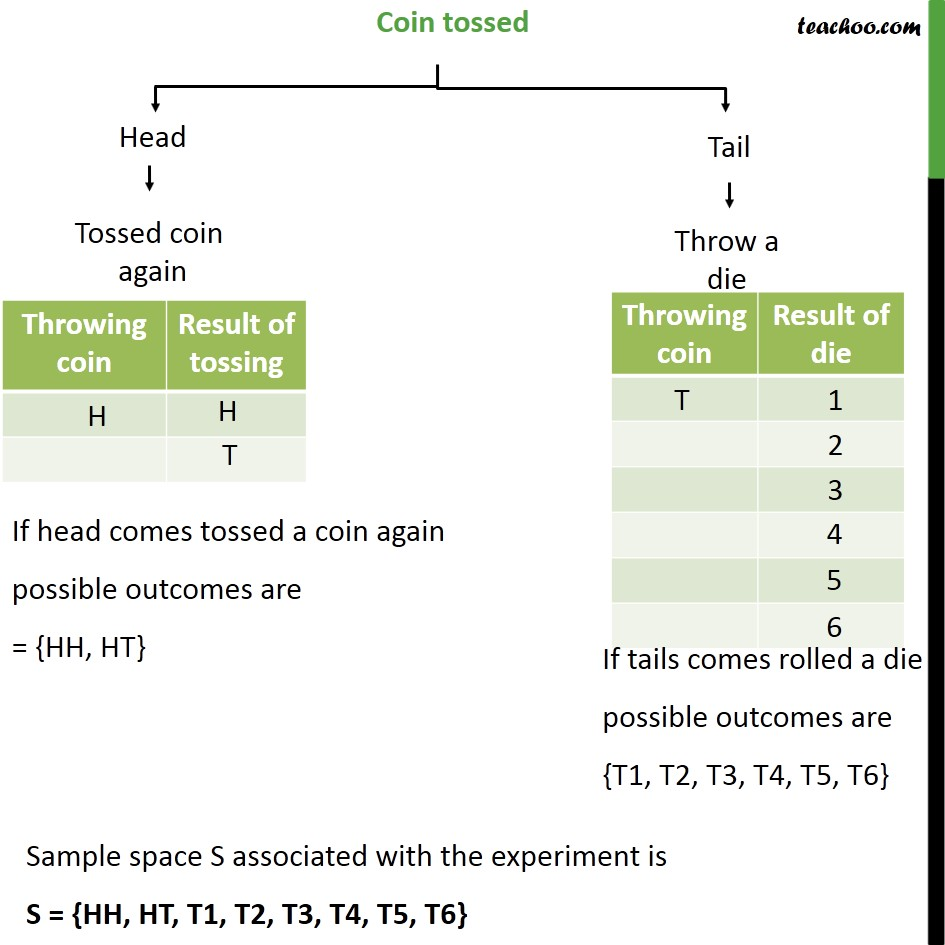1. Chapter 16 Class 11 Probability
2. Concept wise
3. Sample Space

Transcript

Ex 16.1, 10 An experiment consists of tossing a coin and then throwing it second time if a head occurs. If a tail occurs on the first toss, then a die is rolled once. Find the sample space. If a coin tossed there can be either head or tail Let head denote by H & tail denote by T Similarly If a die is rolled possible outcomes are 1, 2, 3, 4, 5, & 6 Sample space S associated with the experiment is S = {HH, HT, T1, T2, T3, T4, T5, T6}

Sample Space